Test Description

## 10 Questions MCQ Test International Mathematics Olympiad (IMO) for Class 10 | Math Olympiad Test: Quadratic Equations- 3

 1 Crore+ students have signed up on EduRev. Have you?

### If x =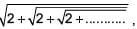then _______.

Detailed Solution for Math Olympiad Test: Quadratic Equations- 3 - Question 1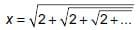...(i)
Squaring both sides of (1), we get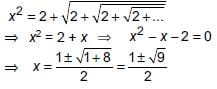Since x cannot be negative, therefore, neglect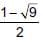Thus, x = 2

### The roots of ax2 + bx + c = 0, a ≠ 0 are real and unequal, if b2 – 4ac is _______.

Detailed Solution for Math Olympiad Test: Quadratic Equations- 3 - Question 2

Given equation is ax2 + bx + c = 0
Roots are real and unequal, if b2 – 4ac > 0

### If one root of the equation a ( b – c ) x2 + b(c – a)x + c(a – b) = 0 is 1, then the other root is ___.

Detailed Solution for Math Olympiad Test: Quadratic Equations- 3 - Question 3

Given equation is a(b – c)x2 + b(c – a)x + c(a – b) = 0
Let α be the other root, then
Product of roots =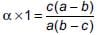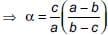If 2 is a root of the equation x2 + bx + 12 = 0 and the equation x2 + bx + q = 0 has equal roots, then q is equal to

Detailed Solution for Math Olympiad Test: Quadratic Equations- 3 - Question 4

Since x = 2 is a root of the equation
x2 + bx + 12 = 0 ⇒ (2)2 + b(2) + 12 = 0
⇒ 2b = –16 ⇒ b = –8
Then, the equation x2 + bx + q becomes
x2 – 8x + q = 0 ...(1)
Since (1) has equal roots ⇒ b2 – 4ac = 0
⇒ (–8)2 – 4(1)q = 0 ⇒ q = 16

One of the two students, while solving a quadratic equation in x, copied the constant term incorrectly and got the roots 3 and 2. The other copied the constant term and coefficient of x2 correctly as –6 and 1 respectively. The correct roots are ____.

Detailed Solution for Math Olympiad Test: Quadratic Equations- 3 - Question 5

Let the equation be x2 + ax + b = 0
Its roots are 3 and 2
∴ Sum of roots, 5 = –a
and product of roots, 6 = b
∴ Equation is x2 – 5x + 6 = 0
Now constant term is wrong and it is given that correct constant term is – 6.
∴ x2 – 5x – 6 = 0 is the correct equation.
Its roots are –1 and 6.

If the roots of the equation (a – b)x2 + (b – c)x + (c – a) = 0 are equal. Then _______.

Detailed Solution for Math Olympiad Test: Quadratic Equations- 3 - Question 6

Since the given equation has equal roots.
∴ D = 0
⇒ (b – c)2 – 4(c – a) (a – b) = 0
⇒ 4a2 + b2 + c2 – 4ab + 2bc – 4ac = 0
⇒ (–2a)2 + (b)2 + (c)2 + 2(–2a)(b) + 2bc + 2(–2a)(c) = 0
⇒ (–2a + b + c)2 = 0
⇒ –2a + b + c = 0 ⇒ 2a = b + c

If one of roots of 2x2 + ax + 32 = 0 is twice the other root, then the value of a is ________.

Detailed Solution for Math Olympiad Test: Quadratic Equations- 3 - Question 7

Equation is 2x2 + ax + 32 = 0
Let one root be α, then other would be 2α.
Now, α × 2α = 16 ⇒ α = ±2√2
and α + 2α = –a/2 ⇒ 3α = –a/2 ⇒ 6α = –a
⇒ ±12√2 = −a or a = ±12√2

If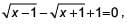then 4x is equal to ________.

Detailed Solution for Math Olympiad Test: Quadratic Equations- 3 - Question 8

Given equation is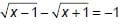...(1)
Rationalising (1), we get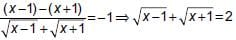...(2)
Adding (1) and (2), we get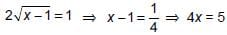Roots of the quadratic equation x2 + x – (a + 1)(a + 2) = 0 are ________.

Detailed Solution for Math Olympiad Test: Quadratic Equations- 3 - Question 9

Given equation is x2 + x – (a + 1)(a + 2) = 0
⇒ x2 + (a + 2)x – (a + 1)x – (a + 1) (a + 2) = 0
⇒ x(x + (a + 2)) – (a + 1)(x + (a + 2)) = 0
⇒ (x – (a + 1))(x + (a + 2)) = 0
⇒ x = (a + 1) or x = – (a + 2)

For what value of a, the roots of t he equation 2x2 + 6x + a = 0, satisfy the condition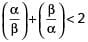(where α and β are the roots of equation).

Detailed Solution for Math Olympiad Test: Quadratic Equations- 3 - Question 10

Given equation is 2x2 + 6x + a = 0
Now,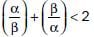⇒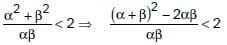⇒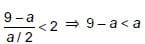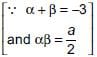⇒ (9/2) < a

## International Mathematics Olympiad (IMO) for Class 10

22 docs|62 tests
 Use Code STAYHOME200 and get INR 200 additional OFF Use Coupon Code